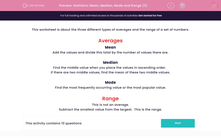# Statistics: Mean, Median, Mode and Range (2)

In this worksheet, students work out the averages and range of a small set of discrete data.This content is premium and exclusive to EdPlace subscribers.Key stage:  KS 2

Curriculum topic:   Maths and Numerical Reasoning

Curriculum subtopic:   Mean, Median, Mode and Range

Difficulty level:#### Worksheet Overview

This worksheet is about the three different types of averages and the range of a set of numbers.

Averages

Mean

Add the values and divide this total by the number of values there are.

Median

Find the middle value when you place the values in ascending order.

If there are two middle values, find the mean of these two middle values.

Mode

Find the most frequently occurring value or the most popular value.

Range

This is not an average.

Subtract the smallest value from the largest.  This is the range.

### What is EdPlace?

We're your National Curriculum aligned online education content provider helping each child succeed in English, maths and science from year 1 to GCSE. With an EdPlace account you’ll be able to track and measure progress, helping each child achieve their best. We build confidence and attainment by personalising each child’s learning at a level that suits them.

Get started